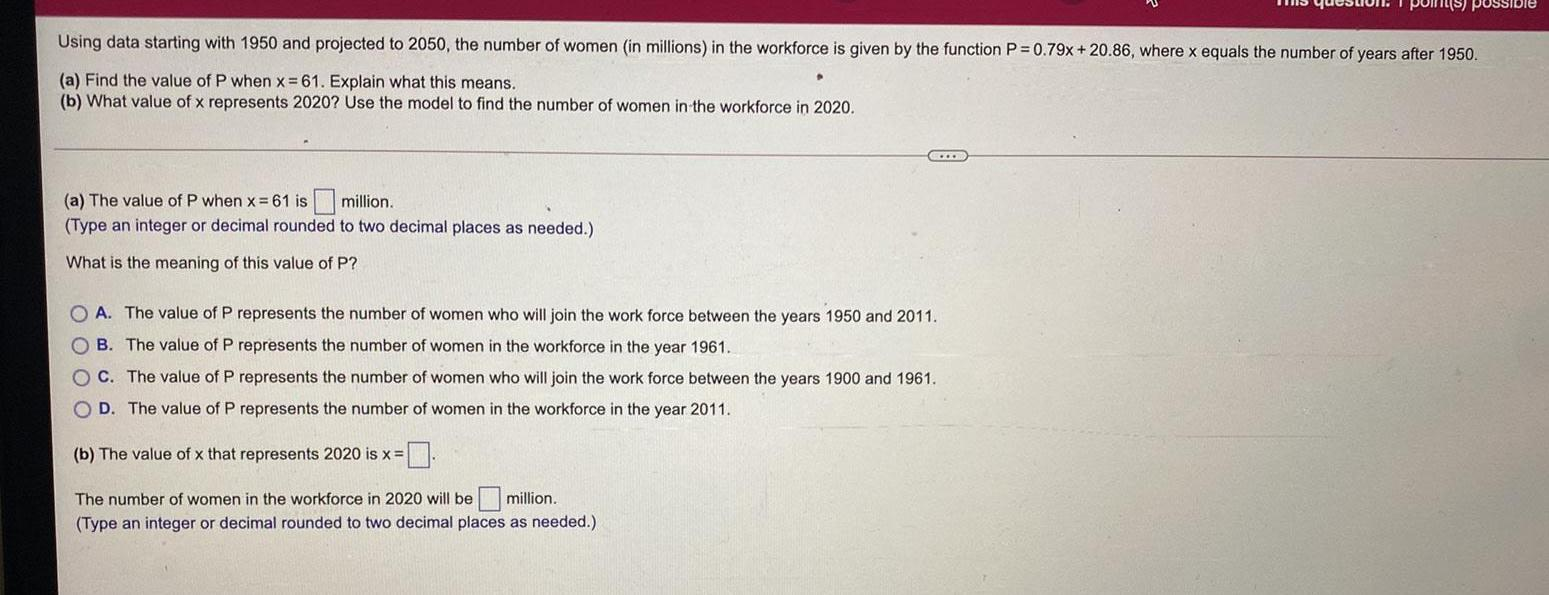Question:

# Using data starting with 1950 and projected to 2050, the

Last updated: 8/9/2022Using data starting with 1950 and projected to 2050, the number of women (in millions) in the workforce is given by the function P = 0.79x+20.86, where x equals the number of years after 1950. (a) Find the value of P when x=61. Explain what this means. (b) What value of x represents 2020? Use the model to find the number of women in the workforce in 2020. (a) The value of P when x=61 is __ million. (Type an integer or decimal rounded to two decimal places as needed.) What is the meaning of this value of P? A. The value of P represents the number of women who will join the work force between the years 1950 and 2011. B. The value of P represents the number of women in the workforce in the year 1961. C. The value of P represents the number of women who will join the work force between the years 1900 and 1961. D. The value of P represents the number of women in the workforce in the year 2011. (b) The value of x that represents 2020 is x = The number of women in the workforce in 2020 will be million. (Type an integer or decimal rounded to two decimal places as needed.).